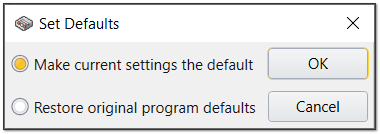The Rocscience International Conference 2021 Proceedings are now available. Read Now

# Solver Options

## Analysis Type

The Analysis Type refers to the interaction between fluid pore pressure and solid stresses and deformation.

## Solver Type

The Solver Type determines how Compute solves the matrix representing the system of equations defined by your model. Two methods are available:

The formulation of boundary element is based on the nodes on the surface of geometry. In traditional approach, in order to obtain the equilibrium of the model, it is required to find the relation between all the nodes on the discretized boundary, which forms a full (not sparse) coefficient matrix. According to this fact, it is required to store the matrix in ram (for large matrixes in both ram and the disc) and then solve the system of equations. There are two methods used in EX3 to solve the equations using traditional boundary element:

• Direct
• Iterative

#### Direct

Direct solver would use the LU decomposition of the matrix to solve the system of equations. The calculation cost of this method is n3 where n is the dimensions of the matrix. This method is not recommended for large models since the memory is not enough to do the operation of decomposition.

#### Iterative

Iterative solver type is used for larger and more complex models with large memory requirement. You can enter advanced option such as maximum number of iteration and tolerance for convergence (higher number of iteration and lower tolerance may improve convergence in most cases but this may not be always the case, so you should be aware of these parameters before using them). Noting that EX3 uses generalized minimum residual (GMRES) method.

### FAST MULTIPLE METHOD (FMM)

This is an efficient method for solving large-scale 3D elastoplastic analysis based on the indirect boundary integral fictitious formulation. This method significantly improves computation time and memory usage, by evaluation integrals through complex-variables asymptotic approximations, similar to Taylor expansions, around the so-called “poles”. The Fast Multipole Method was introduced by Rokhlin (1985) for the solution of the classical 2D potential problem, and it was named as one of the top ten numerical algorithms of the 20th century.

### PRE-CONDITIONED SOLVER

The preconditioned GMRES solver may significantly increase the performance of the engine in most scenarios. A potential optimal performance is achieved by choosing an optimal combination of the scaling limits and the damping parameter. We recommend the default settings of:

damping parameter = 1, upper scaling limit = 1, lower scaling limit = 0.1.

Note: You can change number of load steps to be either Auto or Fixed. We usually recommend users to use auto, but you can define fixed number of load steps depending on the problem.
Note: this option is available only for the Traditional Method

## Set Defaults

To save time, users can set the default Solver Options for future projects by clicking on the Default option.